# Lists CS 5010 Program Design Paradigms Bootcamp Lesson

• Slides: 27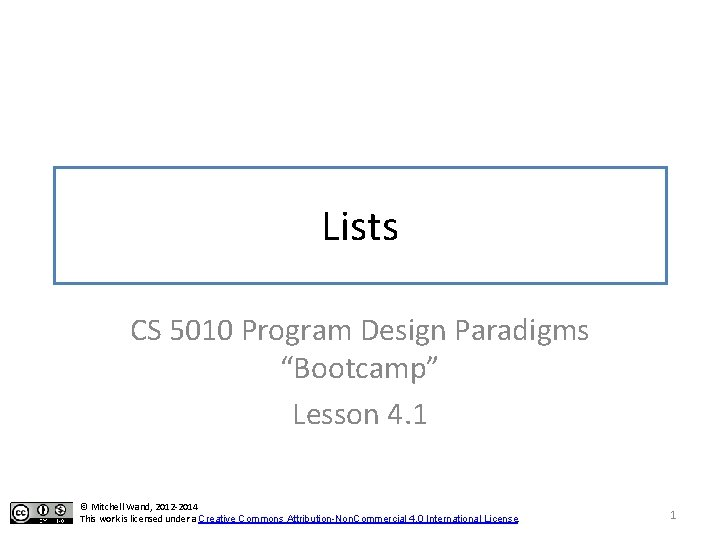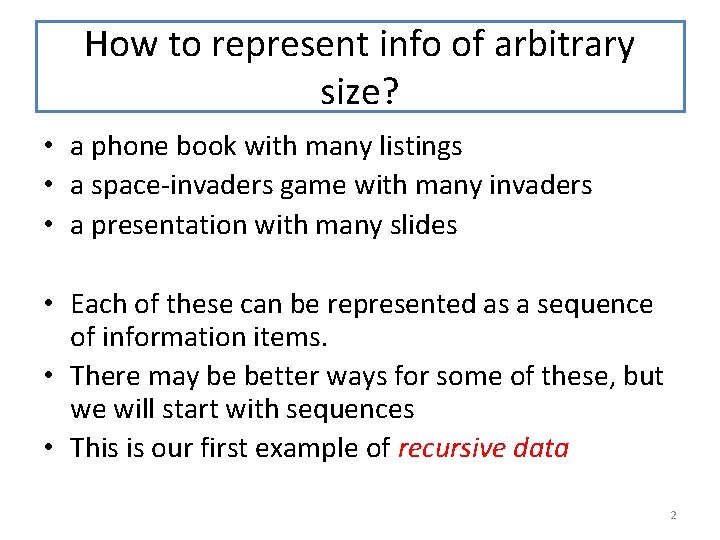How to represent info of arbitrary size? • a phone book with many listings • a space-invaders game with many invaders • a presentation with many slides • Each of these can be represented as a sequence of information items. • There may be better ways for some of these, but we will start with sequences • This is our first example of recursive data 2Data Representations Design Strategies Basics Combine simpler functions Mixed Data Use a template Recursive Data Module 04 Generalization Over Constants Over Expressions Divide into Cases Functional Data Objects & Classes Stateful Objects Over Contexts Call a more general function Communicate via State Over Data Representations Over Method ImplementationsOutline for the rest of this week • The arithmetic of lists • Using the list template • Lists of Structures 4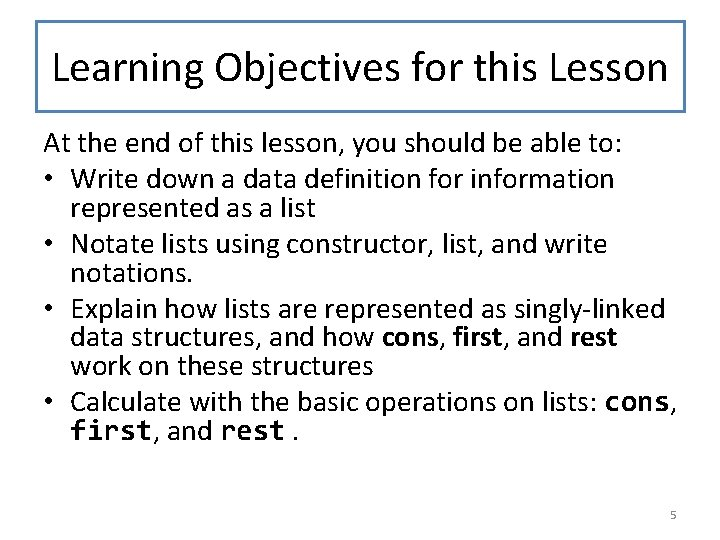Learning Objectives for this Lesson At the end of this lesson, you should be able to: • Write down a data definition for information represented as a list • Notate lists using constructor, list, and write notations. • Explain how lists are represented as singly-linked data structures, and how cons, first, and rest work on these structures • Calculate with the basic operations on lists: cons, first, and rest. 5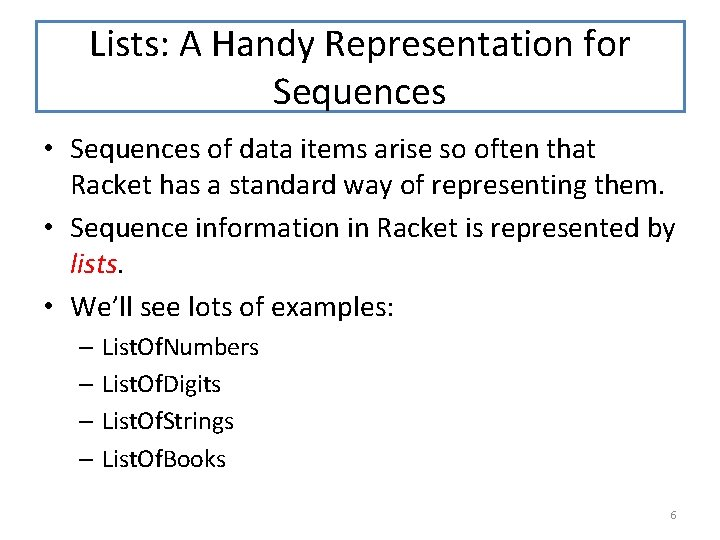Lists: A Handy Representation for Sequences • Sequences of data items arise so often that Racket has a standard way of representing them. • Sequence information in Racket is represented by lists. • We’ll see lots of examples: – List. Of. Numbers – List. Of. Digits – List. Of. Strings – List. Of. Books 6Lists of Numbers A List of Numbers (LON) is one of: -- empty cons is built into Racket. -- (cons Number LON) We don’t need a definestructure for it. List data is a kind of mixed data. Just as we did in our previous data definitions, the data definitions for lists shows the constructor for each case. Here we have two constructors: the constant empty and the function cons. A list of numbers (or "LON") is either empty or the value built by applying cons to a number and another LON. There’s no interpretation here because these lists don’t mean anything (yet). They do not refer to any realworld information. 7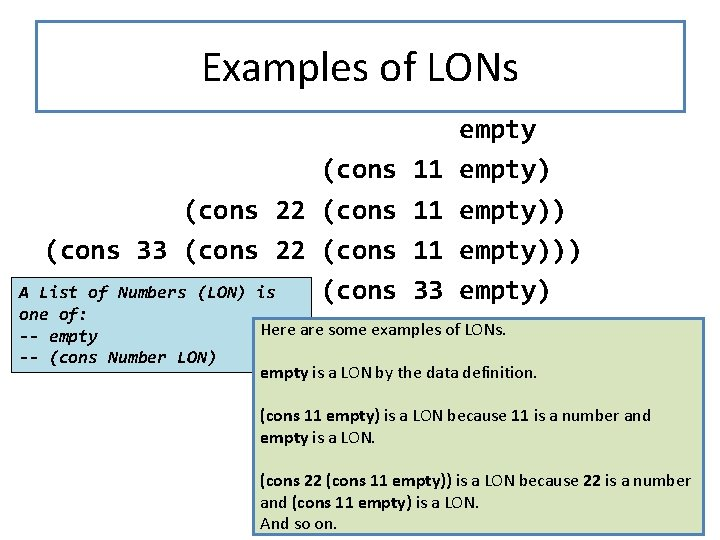Examples of LONs (cons 22 (cons 33 (cons 22 (cons List of Numbers (LON) is (cons A one of: -- empty -- (cons Number LON) 11 11 11 33 empty))) empty) Here are some examples of LONs. empty is a LON by the data definition. (cons 11 empty) is a LON because 11 is a number and empty is a LON. (cons 22 (cons 11 empty)) is a LON because 22 is a number and (cons 11 empty) is a LON. 8 And so on.Lists of Digits A Digit is one of "0" | "1" | "2" |. . . | "9" A List of Digits (LOD) is one of: -- empty Let's do it again, this time with digits. -- (cons Digit LOD) We define a Digit to be one of the strings "0", "1", etc. , through "9". A List of Digits (LOD) is either empty or the cons of a Digit and a List of Digits. 9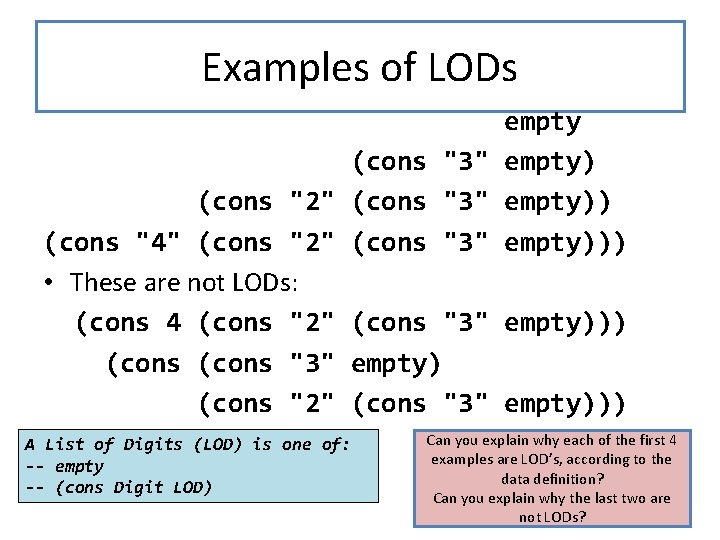Examples of LODs empty (cons "3" empty) (cons "2" (cons "3" empty)) (cons "4" (cons "2" (cons "3" empty))) • These are not LODs: (cons 4 (cons "2" (cons "3" empty))) (cons "3" empty) (cons "2" (cons "3" empty))) A List of Digits (LOD) is one of: -- empty -- (cons Digit LOD) Can you explain why each of the first 4 examples are LOD’s, according to the data definition? Can you explain why the last two are 10 not LODs?Lists of Books A Book is a (make-book. . . ). A List of Books (LOB) is one of: -- empty -- (cons Book LOB) We can build lists of more complicated data items. Imagine we had a data definition for Book. Then we can define a List of Books in the same way as we did for lists of numbers or lists of digits: a List of Books is either empty or the cons of a Book and a List of Books. 11Examples of LOBs (define book 1 (make-book. . . )) (define book 2 (make-book. . . )) (define book 3 (make-book. . . )) empty (cons book 1 empty) (cons book 2 (cons book 1 empty)) • Not a LOB: (Why? ) (cons 4 (cons book 2 (cons book 1 empty)) A List of Books (LOB) is one of: -- empty -- (cons Book LOB) 12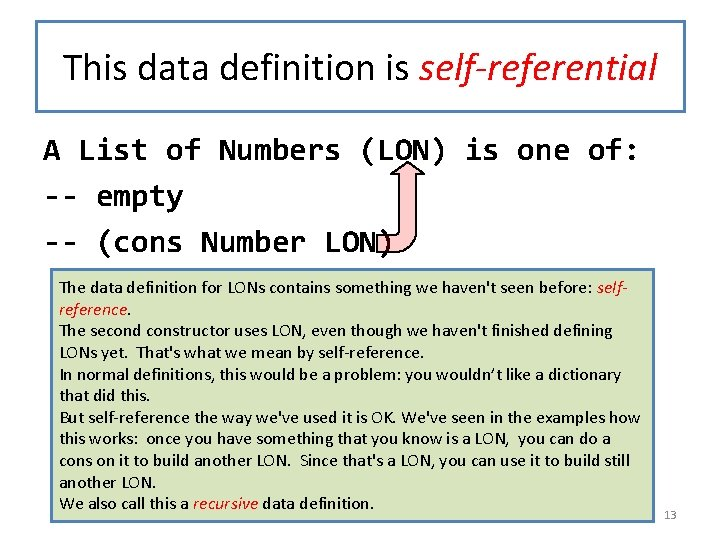This data definition is self-referential A List of Numbers (LON) is one of: -- empty -- (cons Number LON) The data definition for LONs contains something we haven't seen before: selfreference. The second constructor uses LON, even though we haven't finished defining LONs yet. That's what we mean by self-reference. In normal definitions, this would be a problem: you wouldn’t like a dictionary that did this. But self-reference the way we've used it is OK. We've seen in the examples how this works: once you have something that you know is a LON, you can do a cons on it to build another LON. Since that's a LON, you can use it to build still another LON. We also call this a recursive data definition. 13This one is self-referential, too A Digit is one of "0" | "1" | "2" |. . . | "9" A List of Digits (LOD) is one of: -- empty -- (cons Digit LOD) 14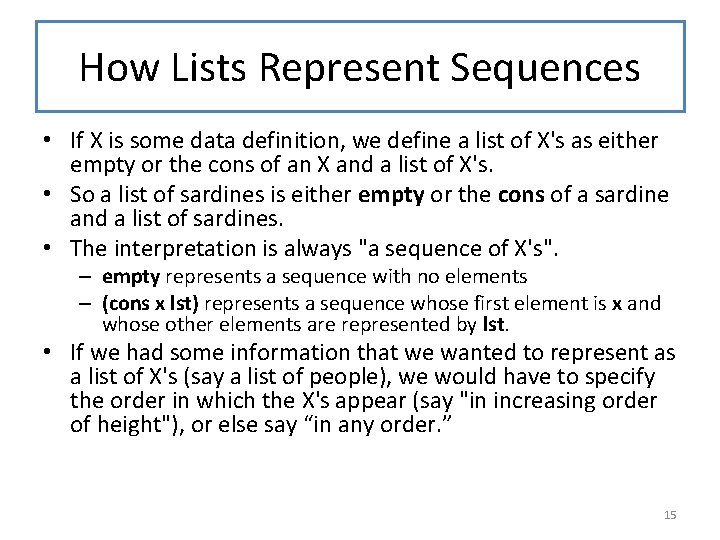How Lists Represent Sequences • If X is some data definition, we define a list of X's as either empty or the cons of an X and a list of X's. • So a list of sardines is either empty or the cons of a sardine and a list of sardines. • The interpretation is always "a sequence of X's". – empty represents a sequence with no elements – (cons x lst) represents a sequence whose first element is x and whose other elements are represented by lst. • If we had some information that we wanted to represent as a list of X's (say a list of people), we would have to specify the order in which the X's appear (say "in increasing order of height"), or else say “in any order. ” 15The General Pattern A List. Of. X is one of -- empty interp: a sequence of X's with no elements -- (cons X List. Of. X) interp: (cons x lst) represents a sequence of X's whose first element is x and whose other elements are represented by lst. 16List Notation • There are several ways to write down lists. • We've been using the constructor notation, since that is the most important one for use in data definitions. • The second most important notation we will use is list notation. In Racket, you can get your output in this notation by choosing the language "Beginning Student with List Abbreviations". • Internally, lists are represented as singly-linked lists. • On output, lists may be notated in write notation. 17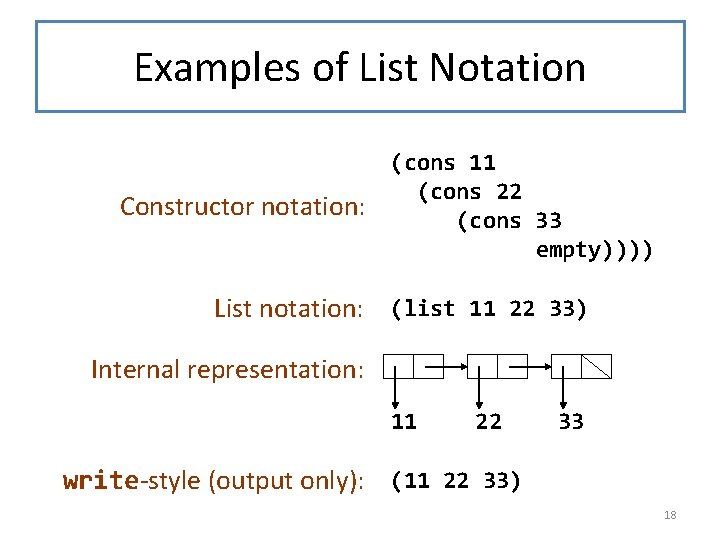Examples of List Notation Constructor notation: (cons 11 (cons 22 (cons 33 empty)))) List notation: (list 11 22 33) Internal representation: 11 22 33 write-style (output only): (11 22 33) 18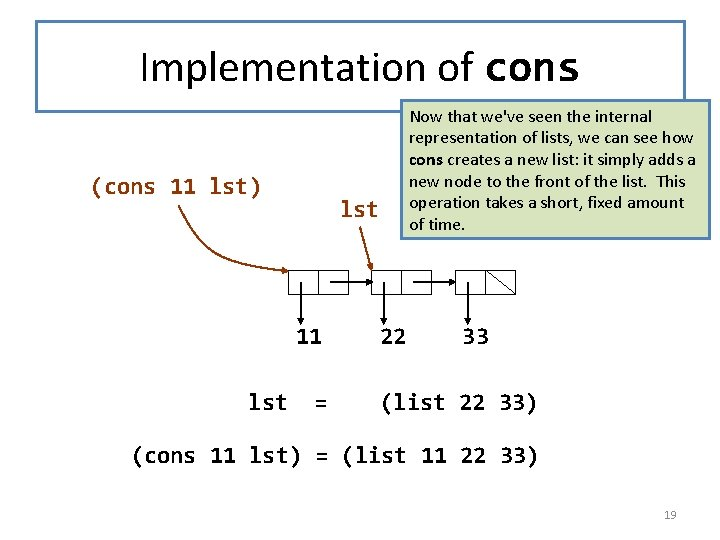Implementation of cons (cons 11 lst) lst 11 lst Now that we've seen the internal representation of lists, we can see how cons creates a new list: it simply adds a new node to the front of the list. This operation takes a short, fixed amount of time. = 22 33 (list 22 33) (cons 11 lst) = (list 11 22 33) 19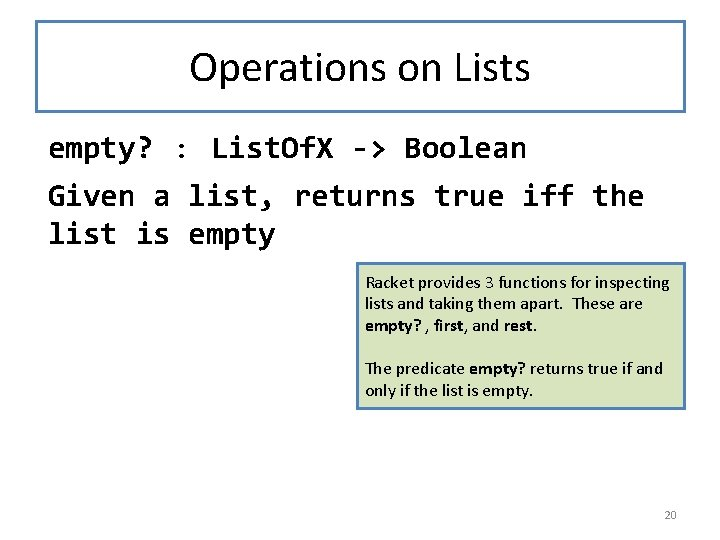Operations on Lists empty? : List. Of. X -> Boolean Given a list, returns true iff the list is empty Racket provides 3 functions for inspecting lists and taking them apart. These are empty? , first, and rest. The predicate empty? returns true if and only if the list is empty. 20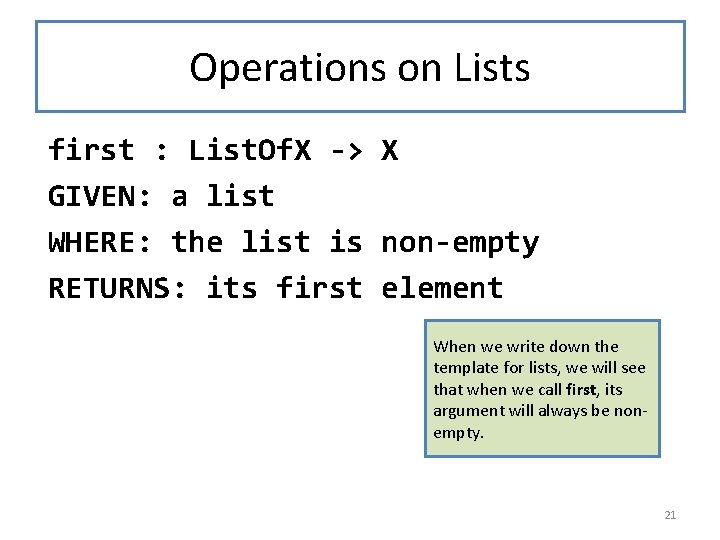Operations on Lists first : List. Of. X -> X GIVEN: a list WHERE: the list is non-empty RETURNS: its first element When we write down the template for lists, we will see that when we call first, its argument will always be nonempty. 21Operations on Lists rest : List. Of. X -> List. Of. X GIVEN: a list WHERE: the list is non-empty RETURNS: the list of all its elements except the first When we write down the template for lists, we will see that when we call rest, its argument will always be nonempty. 22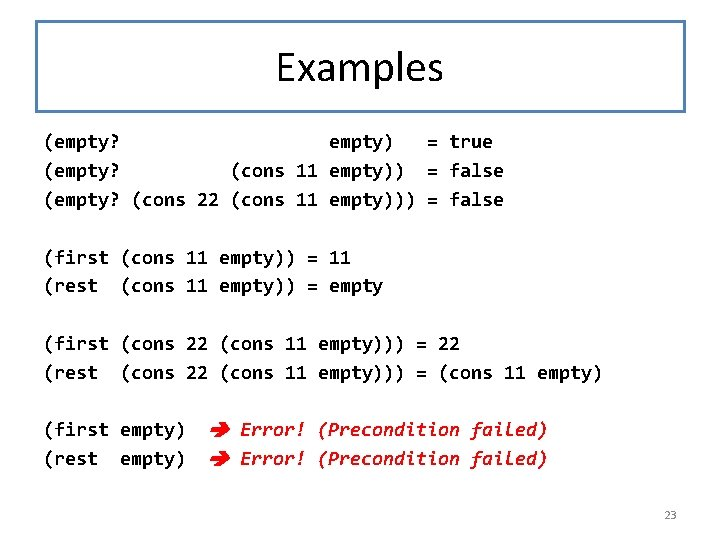Examples (empty? empty) = true (empty? (cons 11 empty)) = false (empty? (cons 22 (cons 11 empty))) = false (first (cons 11 empty)) = 11 (rest (cons 11 empty)) = empty (first (cons 22 (cons 11 empty))) = 22 (rest (cons 22 (cons 11 empty))) = (cons 11 empty) (first empty) (rest empty) Error! (Precondition failed) 23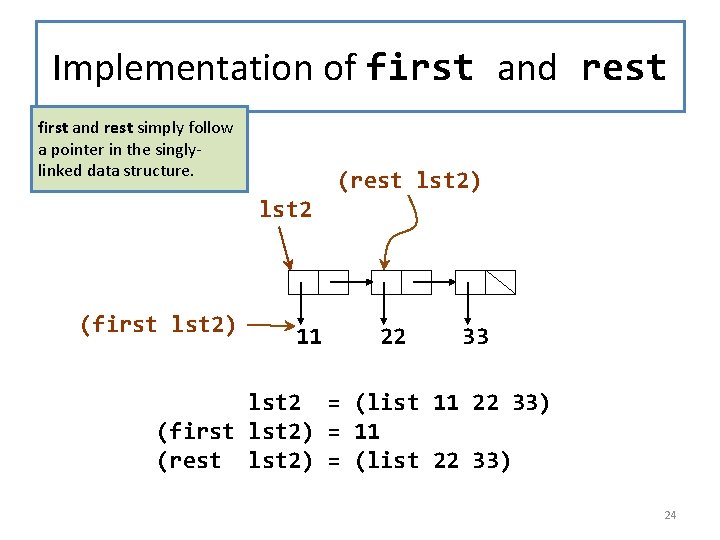Implementation of first and rest simply follow a pointer in the singlylinked data structure. (rest lst 2) lst 2 (first lst 2) 11 22 33 lst 2 = (list 11 22 33) (first lst 2) = 11 (rest lst 2) = (list 22 33) 24Properties of cons, first, and rest (first (cons v l)) = v (rest (cons v l)) = l If l is non-empty, then (cons (first l) (rest l)) = l Here are some useful facts about first, rest, and cons. Can you see why they are true? These facts tell us that if we want to build a list whose first is x and whose rest is lst, we can do this by writing (cons x lst). 25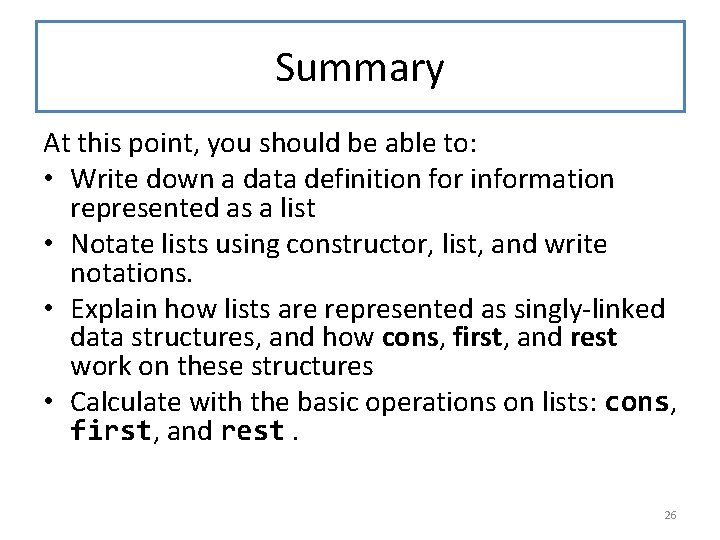Summary At this point, you should be able to: • Write down a data definition for information represented as a list • Notate lists using constructor, list, and write notations. • Explain how lists are represented as singly-linked data structures, and how cons, first, and rest work on these structures • Calculate with the basic operations on lists: cons, first, and rest. 26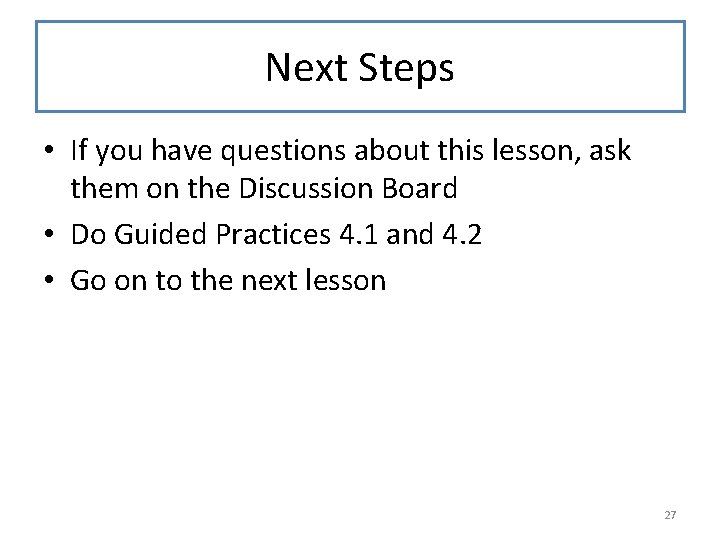Next Steps • If you have questions about this lesson, ask them on the Discussion Board • Do Guided Practices 4. 1 and 4. 2 • Go on to the next lesson 27